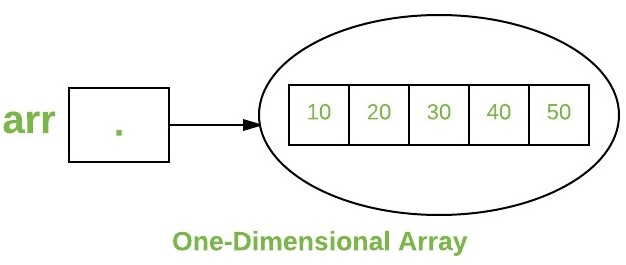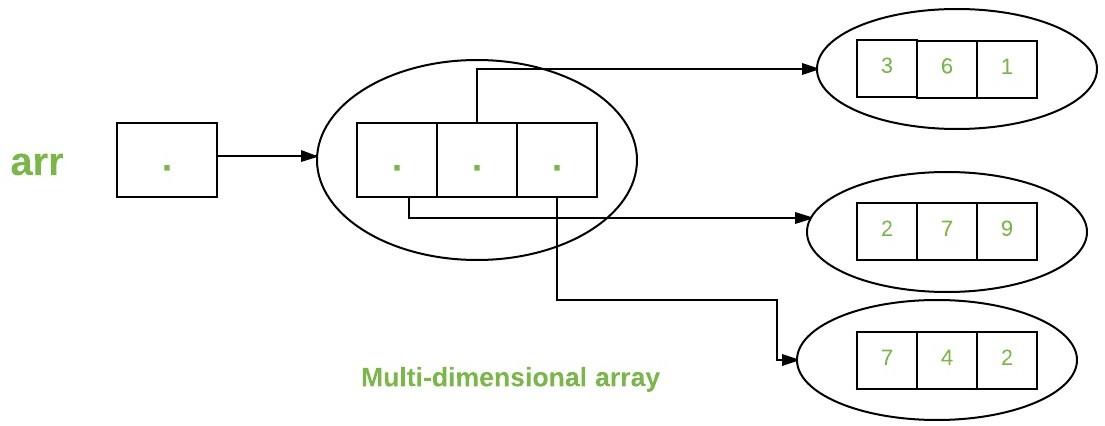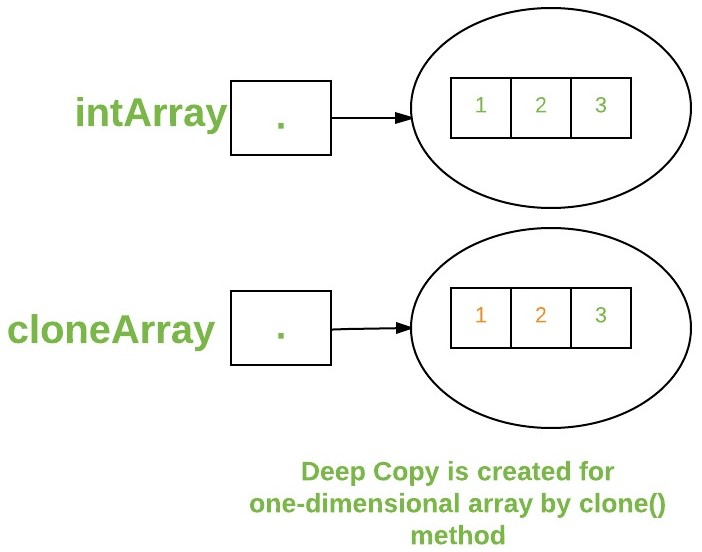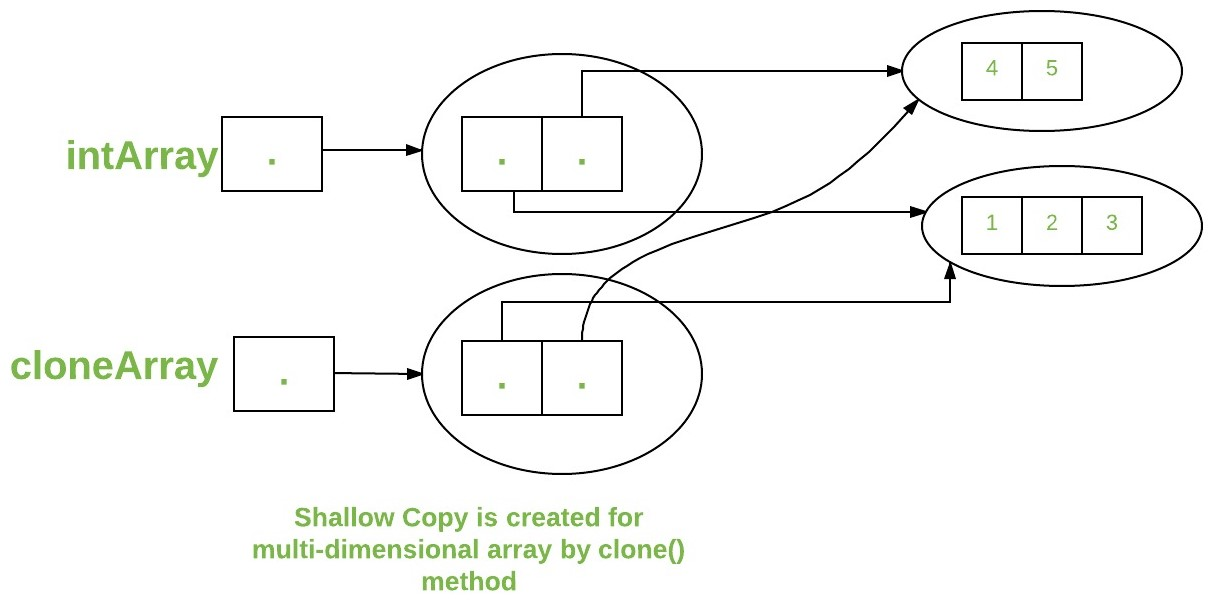# Arrays in Java

## Java Arrays

An array is a group of like-typed variables that are referred to by a common name. Arrays in Java work differently than they do in C/C++. Following are some important points about Java arrays.

• In Java all arrays are dynamically allocated.(discussed below)
• Since arrays are objects in Java, we can find their length using the object property length. This is different from C/C++ where we find length using sizeof.
• A Java array variable can also be declared like other variables with [] after the data type.
• The variables in the array are ordered and each have an index beginning from 0.
• Java array can be also be used as a static field, a local variable or a method parameter.
• The size of an array must be specified by an int or short value and not long.
• The direct superclass of an array type is Object.

Array can contain primitives (int, char, etc.) as well as object (or non-primitive) references of a class depending on the definition of the array. In case of primitive data types, the actual values are stored in contiguous memory locations. In case of objects of a class, the actual objects are stored in heap segment.Creating, Initializing, and Accessing an Array

One-Dimensional Arrays :
The general form of a one-dimensional array declaration is

```type var-name[];
OR
type[] var-name;
```

An array declaration has two components: the type and the name. type declares the element type of the array. The element type determines the data type of each element that comprises the array. Like an array of integers, we can also create an array of other primitive data types like char, float, double, etc. or user-defined data types (objects of a class). Thus, the element type for the array determines what type of data the array will hold.
Example:

```// both are valid declarations
int intArray[];
or int[] intArray;

byte byteArray[];
short shortsArray[];
boolean booleanArray[];
long longArray[];
float floatArray[];
double doubleArray[];
char charArray[];

// an array of references to objects of
// the class MyClass (a class created by
// user)
MyClass myClassArray[];

Object[]  ao,        // array of Object
Collection[] ca;  // array of Collection
// of unknown type
```

Although the first declaration above establishes the fact that intArray is an array variable, no actual array exists. It merely tells the compiler that this variable (intArray) will hold an array of the integer type. To link intArray with an actual, physical array of integers, you must allocate one using new and assign it to intArray.

Instantiating an Array in Java

When an array is declared, only a reference of array is created. To actually create or give memory to array, you create an array like this:The general form of new as it applies to one-dimensional arrays appears as follows:

```var-name = new type [size];
```

Here, type specifies the type of data being allocated, size specifies the number of elements in the array, and var-name is the name of array variable that is linked to the array. That is, to use new to allocate an array, you must specify the type and number of elements to allocate.

Example:

```int intArray[];    //declaring array
intArray = new int;  // allocating memory to array
```

OR

`int[] intArray = new int; // combining both statements in one`

Note :

1. The elements in the array allocated by new will automatically be initialized to zero (for numeric types), false (for boolean), or null (for reference types).
2. Obtaining an array is a two-step process. First, you must declare a variable of the desired array type. Second, you must allocate the memory that will hold the array, using new, and assign it to the array variable. Thus, in Java all arrays are dynamically allocated.

Array Literal

In a situation, where the size of the array and variables of array are already known, array literals can be used.

``` int[] intArray = new int[]{ 1,2,3,4,5,6,7,8,9,10 };
// Declaring array literal```
• The length of this array determines the length of the created array.
• There is no need to write the new int[] part in the latest versions of Java

Accessing Java Array Elements using for Loop

Each element in the array is accessed via its index. The index begins with 0 and ends at (total array size)-1. All the elements of array can be accessed using Java for Loop.

```
// accessing the elements of the specified array
for (int i = 0; i < arr.length; i++)
System.out.println("Element at index " + i +
" : "+ arr[i]);
```

Implementation:

 `// Java program to illustrate creating an array` `// of integers,  puts some values in the array,` `// and prints each value to standard output.` ` ` `class` `GFG ` `{` `    ``public` `static` `void` `main (String[] args) ` `    ``{         ` `      ``// declares an Array of integers.` `      ``int``[] arr;` `         ` `      ``// allocating memory for 5 integers.` `      ``arr = ``new` `int``[``5``];` `         ` `      ``// initialize the first elements of the array` `      ``arr[``0``] = ``10``;` `         ` `      ``// initialize the second elements of the array` `      ``arr[``1``] = ``20``;` `         ` `      ``//so on...` `      ``arr[``2``] = ``30``;` `      ``arr[``3``] = ``40``;` `      ``arr[``4``] = ``50``;` `         ` `      ``// accessing the elements of the specified array` `      ``for` `(``int` `i = ``0``; i < arr.length; i++)` `         ``System.out.println(``"Element at index "` `+ i + ` `                                      ``" : "``+ arr[i]);          ` `    ``}` `}`

Output:

```Element at index 0 : 10
Element at index 1 : 20
Element at index 2 : 30
Element at index 3 : 40
Element at index 4 : 50
```

You can also access java arrays using foreach loopsArrays of Objects

An array of objects is created just like an array of primitive type data items in the following way.

` Student[] arr = new Student; //student is a user-defined class`

The studentArray contains seven memory spaces each of size of student class in which the address of seven Student objects can be stored.The Student objects have to be instantiated using the constructor of the Student class and their references should be assigned to the array elements in the following way.

`Student[] arr = new Student;`
 `// Java program to illustrate creating an array of` `// objects` ` ` `class` `Student` `{` `    ``public` `int` `roll_no;` `    ``public` `String name;` `    ``Student(``int` `roll_no, String name)` `    ``{` `        ``this``.roll_no = roll_no;` `        ``this``.name = name;` `    ``}` `}` ` ` `// Elements of the array are objects of a class Student.` `public` `class` `GFG` `{` `    ``public` `static` `void` `main (String[] args)` `    ``{` `        ``// declares an Array of integers.` `        ``Student[] arr;` ` ` `        ``// allocating memory for 5 objects of type Student.` `        ``arr = ``new` `Student[``5``];` ` ` `        ``// initialize the first elements of the array` `        ``arr[``0``] = ``new` `Student(``1``,``"aman"``);` ` ` `        ``// initialize the second elements of the array` `        ``arr[``1``] = ``new` `Student(``2``,``"vaibhav"``);` ` ` `        ``// so on...` `        ``arr[``2``] = ``new` `Student(``3``,``"shikar"``);` `        ``arr[``3``] = ``new` `Student(``4``,``"dharmesh"``);` `        ``arr[``4``] = ``new` `Student(``5``,``"mohit"``);` ` ` `        ``// accessing the elements of the specified array` `        ``for` `(``int` `i = ``0``; i < arr.length; i++)` `            ``System.out.println(``"Element at "` `+ i + ``" : "` `+` `                        ``arr[i].roll_no +``" "``+ arr[i].name);` `    ``}` `}`

Output:

```Element at 0 : 1 aman
Element at 1 : 2 vaibhav
Element at 2 : 3 shikar
Element at 3 : 4 dharmesh
Element at 4 : 5 mohit
```

What happens if we try to access element outside the array size?

JVM throws ArrayIndexOutOfBoundsException to indicate that array has been accessed with an illegal index. The index is either negative or greater than or equal to size of array.

 `class` `GFG` `{` `    ``public` `static` `void` `main (String[] args)` `    ``{` `        ``int``[] arr = ``new` `int``[``2``];` `        ``arr[``0``] = ``10``;` `        ``arr[``1``] = ``20``;` ` ` `        ``for` `(``int` `i = ``0``; i <= arr.length; i++)` `            ``System.out.println(arr[i]);` `    ``}` `}`

Runtime error

```Exception in thread "main" java.lang.ArrayIndexOutOfBoundsException: 2
at GFG.main(File.java:12)
```

Output:

```10
20
```

Multidimensional Arrays

Multidimensional arrays are arrays of arrays with each element of the array holding the reference of other array. These are also known as Jagged Arrays. A multidimensional array is created by appending one set of square brackets ([]) per dimension. Examples:

```int[][] intArray = new int; //a 2D array or matrix
int[][][] intArray = new int; //a 3D array
```
 `class` `multiDimensional` `{` `    ``public` `static` `void` `main(String args[])` `    ``{` `        ``// declaring and initializing 2D array` `        ``int` `arr[][] = { {``2``,``7``,``9``},{``3``,``6``,``1``},{``7``,``4``,``2``} };` ` ` `        ``// printing 2D array` `        ``for` `(``int` `i=``0``; i< ``3` `; i++)` `        ``{` `            ``for` `(``int` `j=``0``; j < ``3` `; j++)` `                ``System.out.print(arr[i][j] + ``" "``);` ` ` `            ``System.out.println();` `        ``}` `    ``}` `}`

Output:

```2 7 9
3 6 1
7 4 2
```Passing Arrays to Methods

Like variables, we can also pass arrays to methods.For example, below program pass array to method sum for calculating sum of array’s values.

 `// Java program to demonstrate ` `// passing of array to method` ` ` `class` `Test` `{    ` `    ``// Driver method` `    ``public` `static` `void` `main(String args[]) ` `    ``{` `        ``int` `arr[] = {``3``, ``1``, ``2``, ``5``, ``4``};` `         ` `        ``// passing array to method m1` `        ``sum(arr);` `     ` `    ``}` ` ` `    ``public` `static` `void` `sum(``int``[] arr) ` `    ``{` `        ``// getting sum of array values` `        ``int` `sum = ``0``;` `         ` `        ``for` `(``int` `i = ``0``; i < arr.length; i++)` `            ``sum+=arr[i];` `         ` `        ``System.out.println(``"sum of array values : "` `+ sum);` `    ``}` `}`

Output :

```sum of array values : 15
```

Returning Arrays from Methods

As usual, a method can also return an array. For example, below program returns an array from method m1.

 `// Java program to demonstrate ` `// return of array from method` ` ` `class` `Test` `{    ` `    ``// Driver method` `    ``public` `static` `void` `main(String args[]) ` `    ``{` `        ``int` `arr[] = m1();` `         ` `        ``for` `(``int` `i = ``0``; i < arr.length; i++)` `            ``System.out.print(arr[i]+``" "``);` `     ` `    ``}` ` ` `    ``public` `static` `int``[] m1() ` `    ``{` `        ``// returning  array` `        ``return` `new` `int``[]{``1``,``2``,``3``};` `    ``}` `}`

Output:

```1 2 3
```

Class Objects for Arrays

Every array has an associated Class object, shared with all other arrays with the same component type.

 `// Java program to demonstrate ` `// Class Objects for Arrays` ` ` `class` `Test` `{ ` `    ``public` `static` `void` `main(String args[]) ` `    ``{` `        ``int` `intArray[] = ``new` `int``[``3``];` `        ``byte` `byteArray[] = ``new` `byte``[``3``];` `        ``short` `shortsArray[] = ``new` `short``[``3``];` `         ` `        ``// array of Strings` `        ``String[] strArray = ``new` `String[``3``];` `         ` `        ``System.out.println(intArray.getClass());` `        ``System.out.println(intArray.getClass().getSuperclass());` `        ``System.out.println(byteArray.getClass());` `        ``System.out.println(shortsArray.getClass());` `        ``System.out.println(strArray.getClass());` `    ``}` `}`

Output:

```class [I
class java.lang.Object
class [B
class [S
class [Ljava.lang.String;
```

Explanation :

1. The string “[I” is the run-time type signature for the class object “array with component type int“.
2. The only direct superclass of any array type is java.lang.Object.
3. The string “[B” is the run-time type signature for the class object “array with component type byte“.
4. The string “[S” is the run-time type signature for the class object “array with component type short“.
5. The string “[L” is the run-time type signature for the class object “array with component type of a Class”. The Class name is then followed.

Array Members

Now as you know that arrays are object of a class and direct superclass of arrays is class Object.The members of an array type are all of the following:

• The public final field length, which contains the number of components of the array. length may be positive or zero.
• All the members inherited from class Object; the only method of Object that is not inherited is its clone method.
• The public method clone(), which overrides clone method in class Object and throws no checked exceptions.

Cloning of arrays

• When you clone a single dimensional array, such as Object[], a “deep copy” is performed with the new array containing copies of the original array’s elements as opposed to references.
 `// Java program to demonstrate ` `// cloning of one-dimensional arrays` ` ` `class` `Test` `{    ` `    ``public` `static` `void` `main(String args[]) ` `    ``{` `        ``int` `intArray[] = {``1``,``2``,``3``};` `         ` `        ``int` `cloneArray[] = intArray.clone();` `         ` `        ``// will print false as deep copy is created` `        ``// for one-dimensional array` `        ``System.out.println(intArray == cloneArray);` `         ` `        ``for` `(``int` `i = ``0``; i < cloneArray.length; i++) {` `            ``System.out.print(cloneArray[i]+``" "``);` `        ``}` `    ``}` `}`

Output:

```false
1 2 3
```• A clone of a multi-dimensional array (like Object[][]) is a “shallow copy” however, which is to say that it creates only a single new array with each element array a reference to an original element array, but subarrays are shared.
 `// Java program to demonstrate ` `// cloning of multi-dimensional arrays` ` ` `class` `Test` `{    ` `    ``public` `static` `void` `main(String args[]) ` `    ``{` `        ``int` `intArray[][] = {{``1``,``2``,``3``},{``4``,``5``}};` `         ` `        ``int` `cloneArray[][] = intArray.clone();` `         ` `        ``// will print false` `        ``System.out.println(intArray == cloneArray);` `         ` `        ``// will print true as shallow copy is created` `        ``// i.e. sub-arrays are shared` `        ``System.out.println(intArray[``0``] == cloneArray[``0``]);` `        ``System.out.println(intArray[``1``] == cloneArray[``1``]);` `         ` `    ``}` `}`

Output:

```false
true
true
```Last Updated on October 16, 2021 by admin

## Given an array A[] and a number x, check for pair in A[] with sum as xGiven an array A[] and a number x, check for pair in A[] with sum as x

Given an array A[] and a number x, check for pair in A[] with sum

## Find subarray with given sumFind subarray with given sum

Find subarray with given sum Given an unsorted array of nonnegative integers, find a continuous

## Array of Strings in C++Array of Strings in C++

Array of Strings in C++ (All Different Ways to Create) In C and C++, a

## Multidimensional Arrays in JavaMultidimensional Arrays in Java

Multidimensional Arrays in Java Multidimensional Arrays can be defined in simple words as array of

## Merge two sorted arraysMerge two sorted arrays

Merge two sorted arrays Given two sorted arrays, the task is to merge them in

## Write a program to reverse an array or stringWrite a program to reverse an array or string

Program to reverse an array or string Given an array (or string), the task is

## Program to find largest element in an arrayProgram to find largest element in an array

Program to find largest element in an array Given an array, find the largest element

## Largest Sum Contiguous SubarrayLargest Sum Contiguous Subarray

Largest Sum Contiguous Subarray Write an efficient program to find the sum of contiguous subarray